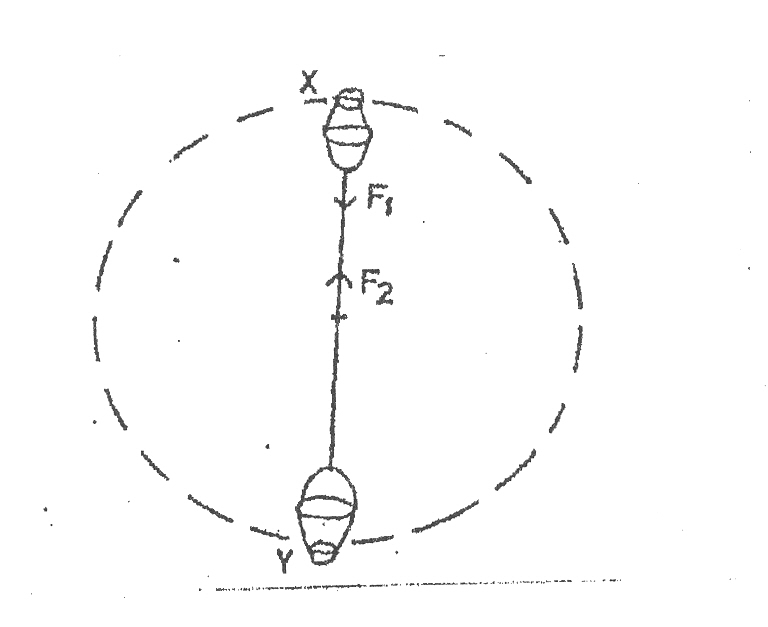Home Technical Mathematics Languages Science Social Science Art Literature Arabic Islamic Studies C.R.K History MusicVisual Art Clothing/Textile Home Management Shorthand
 Physics Paper 2, Nov/Dec. 2009
 Questions: 1 2 3 4 5 6 7 8 9 10 11 12 13 14 15 Main
Weakness/Remedies
Strength

Question 11
Part II:      Candidates were required to answer any five questions from this part.

(a)        State the energy transformations that take place when:
(i)         an electric current is used to drive a crane;
(ii)        an electric bulb is switched on.

(b)        Explain why roads are banked at the bends.

(c)        DiagramA bucket filled with water is tied to a piece of string and whirled in a vertical plane as illustrated in the diagram above.

(i)         Identify the forces F1  and F2.
(ii)        At which position X or Y will the bucket be heavier?  Explain.
(iii)       Give the reason why the water does not pour out at X.

(d)  An electric car of mass 600 kg and efficiency 75% moves up a hill at a constant speed in 80 seconds.  If the hill is 80m long and 5 m high and the car operates on a 36 volts battery, calculate the current supplied by the battery.
[g = 10 ms_2]

_____________________________________________________________________________________________________
Observation

Part (a) was well attempted.  However, some candidates exhibited poor knowledge of the working principle of a filament bulb.  Candidates taught the bulb will produce light before heat, whereas the filament if heated before it glows produces light.

Part (b) was fairly attempted.  However, some candidates were stating why roads were banked at the bends rather than explaining why roads were banked at the bends.

In part (c), candidates were able to identify the forces F1 and F2 as well as explain the position where the bucket is heavier.  However, most responding candidates failed to give the correct reason why the water does not pour out at position X.

In part (d), most candidates cannot relate mechanical energy to electrical energy hence were unable to calculate the current supplied by the battery.  This showed poor knowledge of conversion of energies.

The expected answers are as follows:

(a)        (i)         Electric energy  →   mechanical energy   →   potential energy

OR   Electrical energy  →  mechanical energy

(ii)        Electric energy  →  heat energy  →  light energy

(b)     At unbanked roads the frictional force required to keep a vehicle in a circular
path is not sufficient.  Banking provides an additional force due to the normal reaction on the wheels to prevent the vehicle from slipping.

(c)     (i)         F1  =  centripetal force
F2  =  reactional/centrifugal force

1. The bucket is heavier at position Y.

This is due to the direction of the centripetal force acting.

At position X, weight  =  mv2   -  mg
r

At position Y, weight  =  mv2     +  mg
r
(iii)       The weight acts in the opposite direction to the tension.
The weight of the water is less than the centrifugal force.

1. Efficiency  = Power output

Power input

But Power output  =  force x distance
Time

Po    =   600  x 10  x 5.0
80

=   375 W  =  75 P1
100

Power input    =   100 x 375    =   500W
75

P1   =   IV
I   =  P1  V   =  500    =   13.9A
36